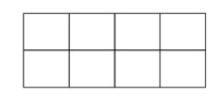# GATE | GATE-CS-2016 (Set 2) | Question 9

• Difficulty Level : Hard
• Last Updated : 03 Oct, 2017

In a 2 × 4 rectangle grid shown below, each cell is a rectangle. How many rectangles can be observed in the grid?(A) 21
(B) 27
(C) 30
(D) 36

Explanation: To form a rectangle, we must choose two horizontal sides and two vertical sides. So, number of rectangles we can form is mC2 * nC2.

Since there are three horizontal lines, we can choose the horizontal sides in 3C2 ways. Since there are five vertical lines, we can choose the vertical sides in 5C2 ways.
The number of rectangles we can form is

= 5C2 * 3C2
= {(5*4)/2}*{(3*2)/2}
= 10*3
= 30

So, option (C) is correct.

Quiz of this Question

My Personal Notes arrow_drop_up